# Checking for and handling autocorrelation

#### 15 March 2016

ACF functions are used for model criticism, to test if there is structure left in the residuals. An important prerequisite is that the data is correctly ordered before running the regression models. If there is structure in the residuals of a GAMM model, an AR1 model can be included to reduce the effects of this autocorrelation.

There are basically two methods to reduce autocorrelation, of which the first one is most important:

1. Improve model fit. Try to capture structure in the data in the model. See the vignette on model evaluation on how to evaluate the model fit: vignette("evaluation", package="itsadug").

2. If no more predictors can be added, include an AR1 model. By including an AR1 model, the GAMM takes into account the structure in the residuals and reduces the confidence in the predictors accordingly.

## How to include an AR1 model

1. First mark the start of each time series as TRUE, and all other data points as FALSE. For measures that develop over time this means typically marking the start of each participant-trial combination. For behavioral or response measures, such as reaction times, this means typically marking the first trial of each participant.

2. Determine the value for the autocorrelation coefficient rho.

## Example

library(itsadug)
library(mgcv)
data(simdat)
# add missing values to simdat:
simdat[sample(nrow(simdat), 15),]$Y <- NA #### Mark start of time series and determine rho In this data, for each individual subject each trial is a unique time series of at most 100 measurements. Mark the start of each time series using the function start_event: simdat <- start_event(simdat, column="Time", event=c("Subject", "Trial"), label.event="Event") head(simdat) ## Group Time Trial Condition Subject Y Event start.event ## 1 Adults 0.00000 -10 -1 a01 0.7554469 a01.-10 TRUE ## 2 Adults 20.20202 -10 -1 a01 2.7834759 a01.-10 FALSE ## 3 Adults 40.40404 -10 -1 a01 1.9696963 a01.-10 FALSE ## 4 Adults 60.60606 -10 -1 a01 0.6814298 a01.-10 FALSE ## 5 Adults 80.80808 -10 -1 a01 1.6939195 a01.-10 FALSE ## 6 Adults 101.01010 -10 -1 a01 2.3651969 a01.-10 FALSE To determine the value of rho, we first have to run a ‘plain’ model to see how strong the residuals are correlated. library(mgcv) # example model: m1 <- bam(Y ~ te(Time, Trial)+s(Subject, bs='re'), data=simdat) Different ways to inspect correlation, they all result in the same picture: par(mfrow=c(1,3), cex=1.1) # default ACF function: acf(resid(m1), main="acf(resid(m1))") # resid_gam: acf(resid_gam(m1), main="acf(resid_gam(m1))") # acf_resid: acf_resid(m1, main="acf_resid(m1)")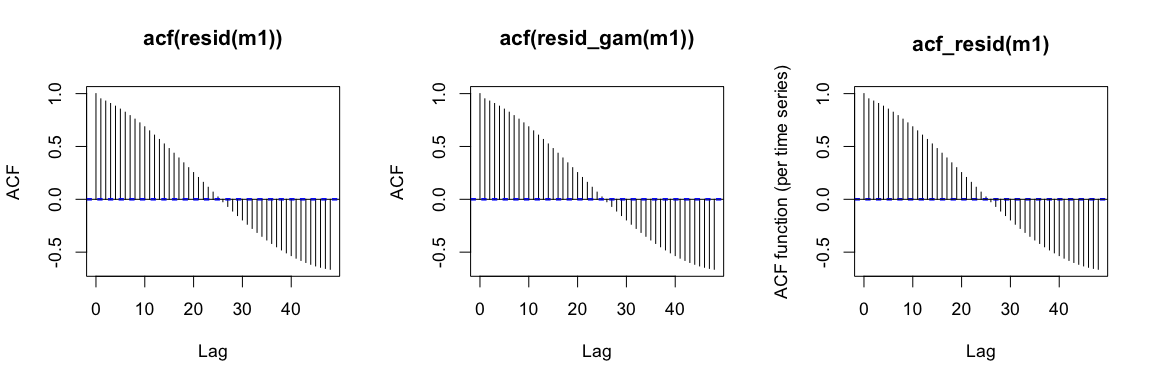Determine the value of lag 1, as indicated by the red dot in the picture below: # we also ask to plot the ACF by specifying plot (FALSE by default): r1 <- start_value_rho(m1, plot=TRUE)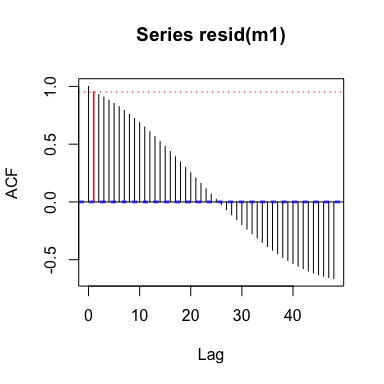The function start_value_rho basically implements the following line: acf(resid(m1), plot=FALSE)$acf
##  0.9506094

#### Run model with AR1 included

Now we have all information to run a model with AR1 model included:

# example model:
m1AR1 <- bam(Y ~ te(Time, Trial)+s(Subject, bs='re'), data=simdat, rho=r1, AR.start=simdat$start.event) ## Inspection of the residuals of an AR1 model By default, the uncorrected residuals are returned. So the default ACF plots of the models m1 and m1AR1 are the same: par(mfrow=c(1,2), cex=1.1) acf(resid(m1)) acf(resid(m1AR1))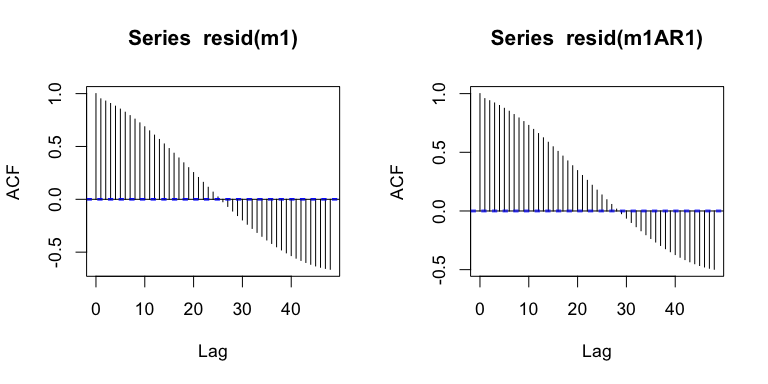Below we list a series of functions that can correct the residuals of a model with AR1 included. #### Function acf_resid Uncorrected versus corrected residuals: par(mfrow=c(1,2), cex=1.1) acf_resid(m1) acf_resid(m1AR1)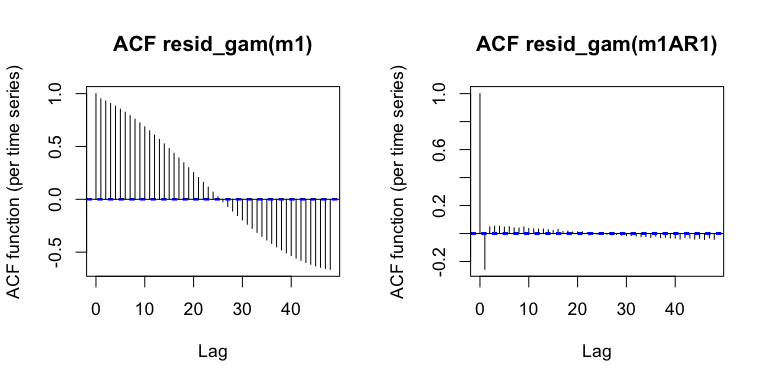Note that the value for rho may have been too high. By default, the data is considered as one single time series. The function can also take into account the different time series, and generate the average ACF over the time series, or examples of ACF functions over individual time series. The argument split_pred specifies the predictor(s) that define the time series. par(mfrow=c(1,3), cex=1.1) acf_resid(m1AR1, split_pred = c("Subject", "Trial")) # alternatively, if the predictors are not found in the model we can use the data: acf_resid(m1AR1, split_pred=list(Subject=simdat$Subject, Trial=simdat$Trial)) # ... or the AR.start information, if provided to the model: acf_resid(m1AR1, split_pred="AR.start")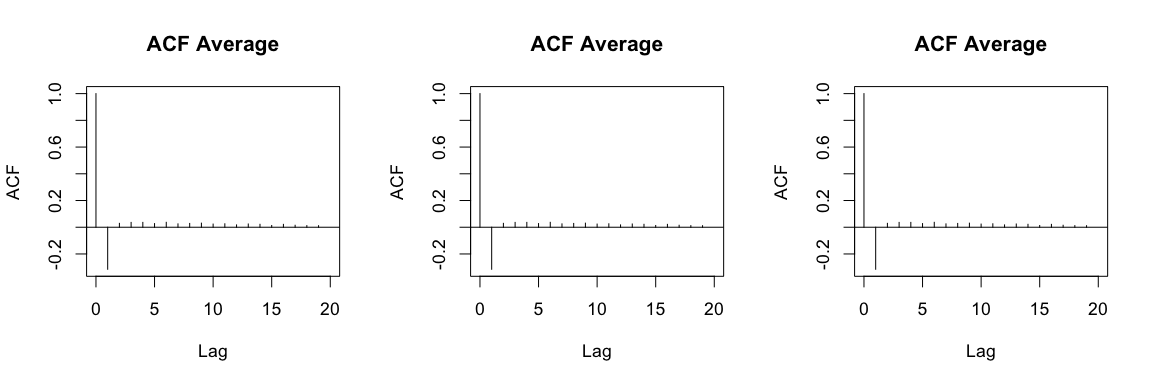The argument n can be used to generate n ACF plots of individual time series. By default examples are chosen that different from each other with respect to the value of lag 1. However, when random is set to TRUE, n randomly selected time series are being plotted. par(cex=1.1) acf_resid(m1AR1, split_pred = c("Subject", "Trial"), n=6) ## Quantiles to be plotted: ## 0% 20% 40% 60% 80% 100% ## -0.6281434 -0.4422719 -0.3640792 -0.2971931 -0.1968636 0.1084543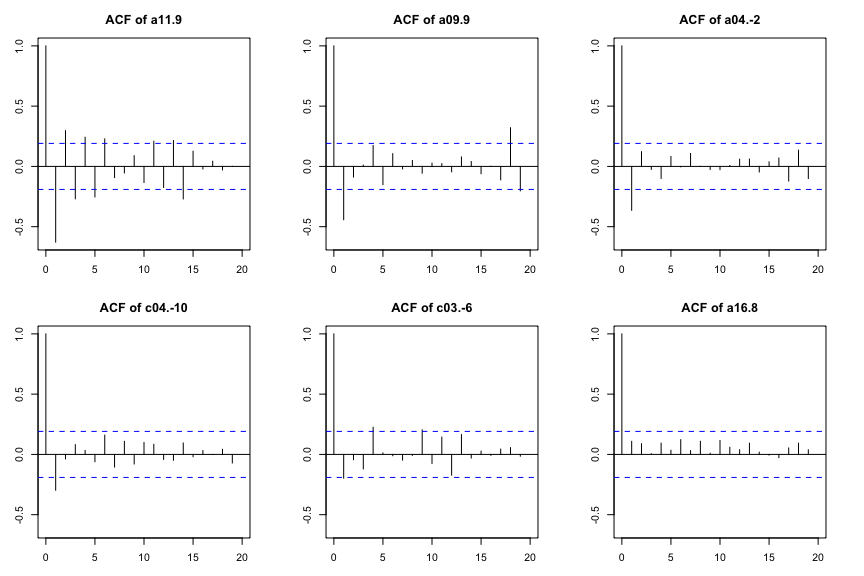The function is basically a wrapper around the functions acf_plot and acf_n_plots, which are explained below. #### Function resid_gam To retrieve the corrected residuals of the model, one could use the function resid_gam. par(mfrow=c(1,2), cex=1.1) # normal residuals: normal.res <- resid(m1AR1) acf(normal.res) # corrected residuals: corrected.res <- resid_gam(m1AR1) acf(corrected.res)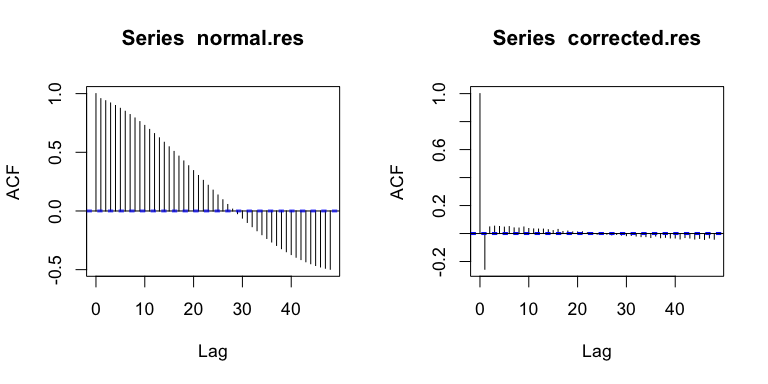Note that the function resid_gam by default does not return NA values (similar to the function resid). In AR1 models there are two sources of missing values: • missing values in the data result in missing values in residuals • missing values are introduced for the first element of each time series As a result, potential problems may arise when storing the residuals in a data.frame. Below the problem and a solution is illustrated: # This will elicit an error: simdat$res.m1 <- resid(m1)
## Error in $<-.data.frame(*tmp*, res.m1, value = c(-1.625494777028, : replacement has 75585 rows, data has 75600 # solution: simdat$res.m1 <- NA
simdat[!is.na(simdat$Y),]$res.m1 <- resid(m1)

# This will generate an error:
simdat$res.m1AR1 <- resid_gam(m1AR1) ## Error in $<-.data.frame(*tmp*, res.m1AR1, value = c(1.43660206297874, : replacement has 74829 rows, data has 75600
# ... and this too!
simdat$res.m1AR1 <- NA simdat[!is.na(simdat$Y),]$res.m1AR1 <- resid_gam(m1AR1) ## Error in $<-.data.frame(*tmp*, res.m1AR1, value = c(1.43660206297874, : replacement has 74829 rows, data has 75585
# solution:
simdat$res.m1AR1 <- NA simdat[!is.na(simdat$Y),]$res.m1AR1 <- resid_gam(m1AR1, incl_na=TRUE) #### Function acf_plot The function acf_plot works on a vector of data, rather than a regression model object – similar to the function acf, but different from acf_resid. The following commands result in the same plot: par(mfrow=c(1,3), cex=1.1) acf(resid_gam(m1AR1)) acf_plot(resid_gam(m1AR1)) acf_resid(m1AR1)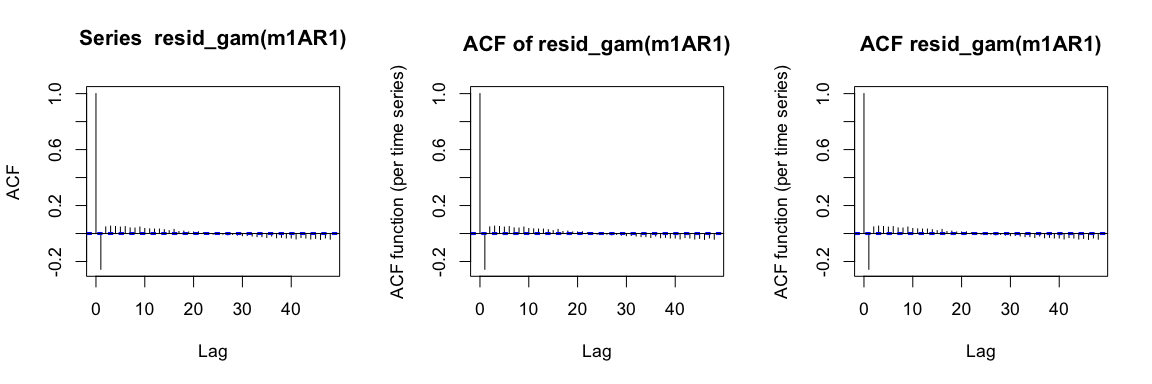The function acf_plot allows for generating different time series and applies a function on the resulting ACF values. The argument split_by expects a list with vectors that define the time series. In contrast with the argument split_pred of the function acf_resid, the argument split_by cannot handle model predictions. The following commands result in the same plot: par(mfrow=c(1,3), cex=1.1) # when using acf_plot one need to remove missing values manually: acf_plot(resid_gam(m1AR1, incl_na = TRUE), split_by=list(Subject=simdat[!is.na(simdat$Y),]$Subject, Trial=simdat[!is.na(simdat$Y),]$Trial)) # ... acf_resid takes care of that automatically: acf_resid(m1AR1, split_pred=c("Subject", "Trial")) # ... also when using a list to identify time series: acf_resid(m1AR1, split_pred=list(Subject=simdat$Subject,
Trial=simdat$Trial))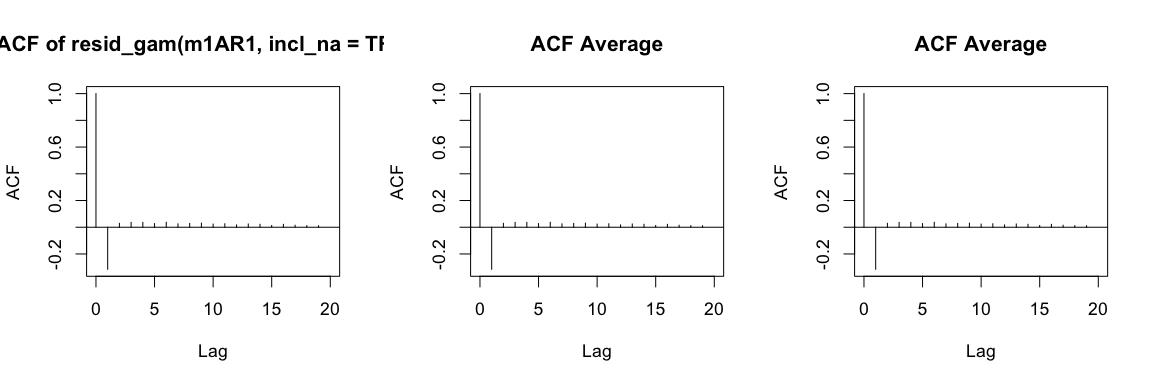So acf_plot is primarily used when the input is not a regression model object – with a regression model object acf_resid is more convenient. Different functions can be applied, including median, sd, and custom functions. tmp <- simdat[!is.na(simdat$Y),]
# default function is mean:
acf.y <- acf_plot(tmp$res.m1, split_by=list(Subject=tmp$Subject, Trial=tmp$Trial), main="ACF with standard deviation") points(as.numeric(names(acf.y)),acf.y, pch=16, cex=.5) # alternatively, we could ask for SE: acf.se <- acf_plot(tmp$res.m1AR1,
split_by=list(Subject=tmp$Subject, Trial=tmp$Trial),
fun=sd, plot=FALSE)
add_bars(as.numeric(names(acf.se)), y=acf.y+acf.se, y0=acf.y-acf.se, col=NA, border=2, width=.5)
legend('topright', legend="sd", fill=NA, border=2, bty='n')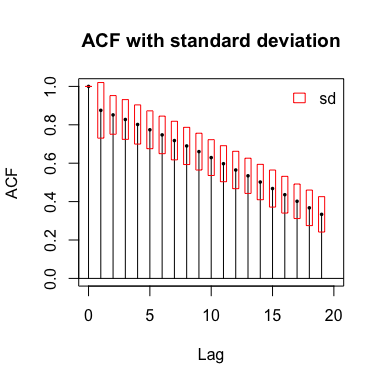#### Function acf_n_plot

The function acf_n_plots calculates an ACF for each event, and can be used to plot multiple examples. These examples are random selected or selected as maximally different on the basis of the lag 1-values of the events.

#### Function derive_timeseries

The function derive_timeseries can be used to extract time series from the model, on the basis of the AR.start information provided to the model. The function only works if the AR.start argument is used.

simdat$Event <- NA simdat[!is.na(simdat$Y),]$Event <- derive_timeseries(m1AR1) str(simdat) ## 'data.frame': 75600 obs. of 10 variables: ##$ Group      : Factor w/ 2 levels "Children","Adults": 2 2 2 2 2 2 2 2 2 2 ...
##  $Time : num 0 20.2 40.4 60.6 80.8 ... ##$ Trial      : int  -10 -10 -10 -10 -10 -10 -10 -10 -10 -10 ...
##  $Condition : int -1 -1 -1 -1 -1 -1 -1 -1 -1 -1 ... ##$ Subject    : Factor w/ 36 levels "a01","a02","a03",..: 1 1 1 1 1 1 1 1 1 1 ...
##  $Y : num 0.755 2.783 1.97 0.681 1.694 ... ##$ Event      : int  1 1 1 1 1 1 1 1 1 1 ...
##  $start.event: logi TRUE FALSE FALSE FALSE FALSE FALSE ... ##$ res.m1     : num  -1.6255 -0.0781 -1.3716 -3.1377 -2.6002 ...
##  \$ res.m1AR1  : num  1.4366 -1.3285 -1.8656 0.3502 0.0388 ...